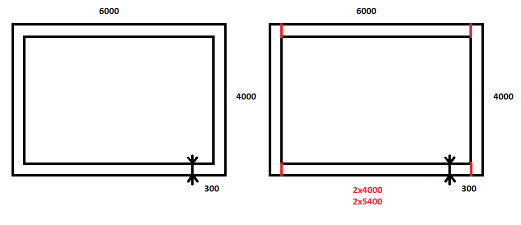# Construction Volume Calculator

The Construction Volume Calculator allows you to calculate the total volume of a construction project by specifying the volume of various prisms (or rectangular cuboids) and subtracting any volumes you wish to exclude.

Simply input the height, width, and length of each prism and indicate whether to add or subtract the volume from the total. You can also add hints to help you keep track of which prism corresponds to which part of your construction project.Example: Let's say you're building a house and you want to calculate the total volume of the walls, minus the volume of the doors. You would input the height, width, and length of each wall as separate prisms, and label them "Wall". Then, you would input the height, width, and length of each door as separate prisms, and label them "Door". For the walls, you would choose to add their volume to the total, while for the doors, you would choose to subtract their volume from the total. You could also add a hint such as "Wall 1", "Wall 2", "Door 1", etc. to help you keep track of which prism corresponds to which part of your construction project.

Note that you should be careful when breaking down structure to figures to avoid double counting of same volumes. The picture below illustrates the problem:Here you need to subtract width for two walls to get correct dimensions. Well, it is simple enough and I hope that the idea is clear.#### Volumes

Length, mmWidth, mmHeight, mmQuantityTypeHint
Items per page:

Digits after the decimal point: 3